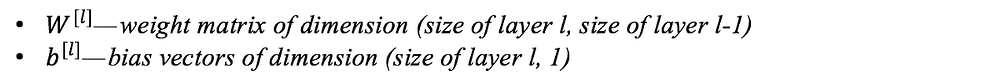# Weight Initialization Techniques in Neural Networks

Source: Deep Learning on Medium

Building even a simple neural network can be confusing task and upon that tuning it to get a better result is extremely tedious. But, the first step that comes in consideration while building a neural network is initialization of parameters, if done correctly then optimization will be achieved in least time otherwise converging to a minima using gradient descent will be impossible.

This article has been written under the assumption that reader is already familiar with the concept of neural network, weight, bias, activation functions, forward and backward propagation etc.

#### Basic notations

Consider a L layer neural network, which has L-1 hidden layers and 1 input and output layer each. The parameters (weights and biases) for layer l are represented as

In this article we’ll have a look at some of the basic initialization practices in use and some improved techniques that must be used in order to achieve better result. Following are some techniques generally practiced to initialize parameters :

• Zero initialization
• Random initialization

#### Zero initialization :

In general practice biases are initialized with 0 and weights are initialized with random numbers, what if weights are initialized with 0 ?

In order to understand this lets consider we applied sigmoid activation function for the output layer .

If all the weights are initialized with 0 , the derivative with respect to loss function is same for every w in W[l], thus all weights have same value in subsequent iterations. This makes hidden units symmetric and continues for all the n iterations i.e. setting weights to 0 does not make it better than a linear model. An important thing to keep in mind is that biases have no effect what so ever when initialized with 0.

`W[l] = np.random.zeros((l-1,l))`

lets consider a neural network with only three hidden layer with ReLu activation function in hidden layers and sigmoid for the output layer.

Using above neural network on dataset “make circles” from sklearn.datasets, result obtained was following :

for 15000 iterations, loss = 0.6931471805599453, accuracy = 50 %

clearly, zero initialization isn’t successful in classification.

#### Random initialization :

Assigning random values to weights is better than just 0 assignment. But there is one thing to keep in my mind is that what happens if weights are initialized high values or very low values and what is a reasonable initialization of weight values.

a) If weights are initialized with very high values the term `np.dot(W,X)+b` becomes significantly higher and if an activation function like sigmoid() is applied, the function maps its value near to 1 where slope of gradient changes slowly and learning takes a lot of time.

b) If weights are initialized with low values it gets mapped to 0, where the case is same as above.

This problem is often referred to as vanishing gradient.

To see this lets see the example we took above but now the weights are initialized with very large values instead of 0 :

`W[l] = np.random.randn(l-1,l)*10`

Neural network is same as earlier, using this initialization on dataset “make circles” from sklearn.datasets, result obatined was following :

for 15000 iterations, loss = 0.38278397192120406, accuracy = 86 %

This solution is better but doesn’t properly fulfill the needs so, lets see a new technique.

#### New Initialization techniques

As we saw above that with large or 0 initialization of weights(W), not significant result is obtained even if we use appropriate initialization of weights it is probable that training process is going to take longer time. There are certain problems associated with it :

a) If model is too large and takes many days to train then what

These were some problems that stood in the path for many years but in 2015, He et al.(2015) proposed activation aware initialization of weights (for ReLu) that was able to resolve this problem. ReLu and leaky ReLu also solves the problem of vanishing gradient.

He initialization : we just simply multiply random initialization with

To see how effective this solution is, lets use the previous dataset and neural network we took for above initialization and results are :

for 15000 iterations, loss =0.07357895962677366, accuracy = 96 %

Surely, this is an improvement over the previous techniques.

There are also some other techniques other than He initialization in use that are comparatively better than old techniques and are used frequently.

Xavier initialization : It is same as He initialization but it is used for tanh() activation function, in this method 2 is replaced with 1.

Some also use the following technique for initialization :

These methods serve as good starting points for initialization and mitigate the chances of exploding or vanishing gradients. They set the weights neither too much bigger that 1, nor too much less than 1. So, the gradients do not vanish or explode too quickly. They help avoid slow convergence, also ensuring that we do not keep oscillating off the minima. There exist other variants of the above, where the main objective again is to minimize the variance of the parameters. Thank you.